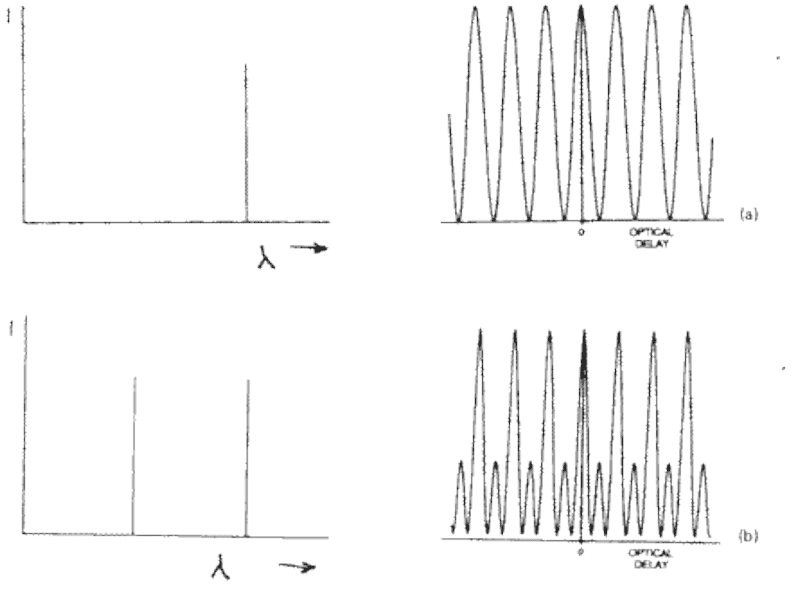# Doubts about Fourier transform of IR spectroscopy

• I
Salmone
I was studying a Michelson interferometer for infrared absorption in Fourier transform and I've found these two images (taken from https://pages.mtu.edu/~scarn/teaching/GE4250/ftir_lecture_slides.pdf ) representing an infrared monochromatic beam of light going into the interferometer and the resulting interferogram which is of course a cosine function and two monochromatic beams of light going into the interferometer which has the resulting interferogram.

With focus to the second interferogram, when the "optical path difference" (OPD from now on) is equal to ##0## of course we have a maximum of the intensity since both beams interfere constructively and when the movable mirror is moved so that the OPD is equal to ##\frac{\lambda_1}{2}## where ##\lambda_1## is the wavelenght of one of the beams, this one interfere desctructively with his part that traveled on the other path eliminating each other and the beam of wavelenght ##\lambda_2## also interfere with his part so that we get an intensity ##\neq 0## but smaller then the first one obtained when ##OPD=0##.

My question is:

At some points in the interferogram we see that total intensity of light is zero, in my opinion we get zero intensity when the movable mirror is placed at a point such that the OPD is equal to ##(n+\frac{1}{2})\lambda## for both beams, but this condition is only possible if ##\lambda_1=n\lambda_2## with ##n## being an integer, is this the only condition in which we get total intensity equal to zero?

Are we always sure that there will be a certain position for the movable mirror such that the total intensity is equal to zero?Homework Helper
At some points in the interferogram we see that total intensity of light is zero, in my opinion we get zero intensity when the movable mirror is placed at a point such that the OPD is equal to (n+1/2)λ for both beams, but this condition is only possible if λ1=nλ2 with n being an integer, is this the only condition in which we get total intensity equal to zero?
Be careful how you use n; inconsistency may lead to confusion. The condition is that OPD = (n+1/2)λ1 and (m+1/2)λ2 simultaneously, where m and n are integers. This implies
λ12 = (2m+1)/(2n+1), i.e. the ratio of two odd integers.
It is also necessary that the intensities of the two beams are equal.
Diagram b appears to show λ1 = 2λ2; in this case the interference pattern would not have zero intensity at any point.

•